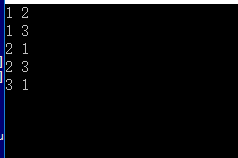`````` #include<stdio.h>
#include<malloc.h>

#define max 10000
int edge={0};
typedef struct _enode
{
//int dis;
int vex;
struct _enode* nextedge;
}enode;

typedef struct _vnode
{
int vex;
enode* firstedge;
}vnode;

typedef struct _pic
{
int vex_num;
int edge_num;
vnode node[max];
}pic;

void find_insert(enode* edge,enode* temp)
{
enode* p;
p=edge;
while(p->nextedge!=NULL)
p=p->nextedge;
p->nextedge=temp;
}

pic* create_pic()
{
int i=0,c1,c2;
enode* node_temp;
pic* picture;
picture=(pic*)malloc(sizeof(pic));
picture->edge_num=5;
picture->vex_num=3;

for(i=1;i<=picture->vex_num;i++)   //you are sb。I just say to you
{
picture->node[i].vex=i;
picture->node[i].firstedge=NULL;
c1=edge[i];
c2=edge[i];
node_temp=(enode*)malloc(sizeof(struct _enode));
node_temp->vex=c2;

if(picture->node[c1].firstedge==NULL)
{
picture->node[c1].firstedge=node_temp;
}
else
{
find_insert(picture->node[c1].firstedge,node_temp);
}
}

return picture;
}

int main(void)
{
int i=0;
pic* picture;
for(i=1;i<=5;i++)
{
scanf("%d",&edge[i]);
scanf("%d",&edge[i]);
}
picture=create_pic();

for(i=1;i<=picture->vex_num;i++)
{
enode* p;
p=(enode*)malloc(sizeof(enode));
if(picture->node[i].firstedge==NULL)
printf("error");
else
{
p=picture->node[i].firstedge;
printf("%d ",picture->node[i].vex);
while(p!=NULL)
{
printf("%d ",p->vex);
p=p->nextedge;
}
}
printf("\n");
}

}
``````3个回答

pic* create_pic()函数中，node_temp中的nextedge未赋初值，导致find_insert函数中判断出错，改一下create_pic()函数：

``````node_temp=(enode*)malloc(sizeof(struct _enode));
node_temp->vex=c2;
node_temp->nextedge=NULL; //加上这句，不然判断会出错
``````